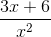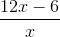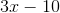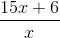# PSAT Math : How to add rational expressions with a common denominator

## Example Questions

### Example Question #1 : Rational Expressions

Simplify.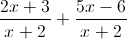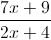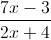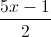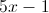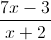Explanation: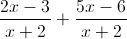Same denominator means you add straight across the numerators, keeping the denominator the same.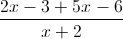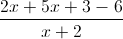### Example Question #41 : Expressions

Simplify.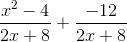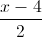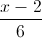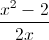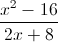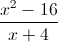Explanation:Check for same Denominator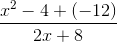Check for GCF or if the expression can be factored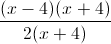After factoring, divide out like terms.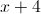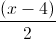### Example Question #1 : How To Add Rational Expressions With A Common Denominator

Simplify the following rational expression: (9x - 2)/(x2) MINUS (6x - 8)/(x2)